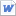검색어 입력폼

# 정진호교수 맨큐의 경제학 14단원

저작시기 2014.07 |등록일 2014.11.23MS 워드 (docx) | 1페이지 | 가격 700원

없음

## 본문내용

This figure shows the The marginal cost curve(MC) and the average variable cost curve(AVC). It also shows the market price(P), which equals marginal revenue(MR) and average revenue(AR). At the quantity Q1, marginal revenue MR1 exceeds marginal cost MC1, so raising production increases profit. At the quantity Q2, Marginal cost MC2 is above marginal revenue MR2, so reducing production increases profit. The profit-maximizing quantity Qmax is found where the horizontal price line intersects the marginal-cost curve.

없음